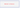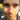### Lanky Dan Blog# Method::reference

January 18, 2017

javajava 8

Method references are a feature of Java 8. They are effectively a subset of Lambda expressions because if a Lambda expression can be used then it might be possible to use a method reference, but not always. They can only be used to call a singular method, which obviously reduces the possible places they can be used unless your code is written to cater for them.

It would be a good idea if you knew the notation for a method reference, in fact you have probably already seen it assuming you read the title. If not then just look below.

``Person::getName``

The example above is the equivalent of writing `person.getName()` where `person` is a instance of `Person`. Let me tell you a bit more about when you can use method references and show some examples as it makes a lot more sense with them.

## Types of method references

Type Syntax Method Reference Lambda expression
Reference to a static method Class::staticMethod `String::valueOf` `s -> String.valueOf(s)`
Reference to an instance method of a particular object instance::instanceMethod `s::toString` `() -> “string”.toString()`
Reference to an instance method of an arbitrary object of a particular type Class:instanceMethod `String::toString` `s -> s.toString()`
Reference to a constructor Class::new `String::new` `() -> new String()`

### Reference to a static method

``````public class StaticMethodReference{
public static void main(String args[]) {
List<Integer> list = Arrays.asList(1, 2, 3, 4, 5, 6, 7, 8, 9, 10);
// Method reference
list.forEach(StaticMethodReference::print);
// Lambda expression
list.forEach(number -> StaticMethodReference.print(number));
// normal
for(int number : list) {
StaticMethodReference.print(number);
}
}

public static void print(final int number) {
System.out.println("I am printing: " + number);
}
}``````

Here it calls the static method `StaticMethodReference.print.`This example is pretty simple, there is a static method and for each element in the list it calls this method using the element as the input.

### Reference to an instance method of a particular object

``````public class ParticularInstanceMethodReference {
public static void main(String args[]) {
final List<Integer> list = Arrays.asList(1, 2, 3, 4, 5, 6, 7, 8, 9, 10);
final MyComparator myComparator = new MyComparator();
// Method reference
Collections.sort(list, myComparator::compare);
// Lambda expression
Collections.sort(list, (a,b) -> myComparator.compare(a,b));
}

private static class MyComparator {
public int compare(final Integer a, final Integer b) {
return a.compareTo(b);
}
}
}``````

Here it calls the instance method `myComparator.compare` where `myComparator` is an particular instance of `MyComparator`.

### Reference to an instance method of an arbitrary object of a particular type

``````public class ArbitraryInstanceMethodReference {
public static void main(String args[]) {
final List<Person> people = Arrays.asList(new Person("dan"), new Person("laura"));
// Method reference
people.forEach(Person::printName);
// Lambda expression
people.forEach(person -> person.printName());
// normal
for (final Person person : people) {
person.printName();
}
}

private static class Person {
private String name;

public Person(final String name) {
this.name = name;
}

public void printName() {
System.out.println(name);
}
}
}``````

This calls the method `Person.getName` for each `Person` object in the list. `Person` is the particular type and the arbitrary object is the instance of `Person` that is used during each loop. This looks very similar to a reference to a static method but the difference is how the object is passed to the method reference. Remember a static reference passes the current object into the method whereas an arbitrary method reference invokes a method onto the current object.

### Reference to a constructor

``````public class ConstructorMethodReference {
public static void main(String args[]) {
final List<Integer> list = Arrays.asList(1, 2, 3, 4, 5, 6, 7, 8, 9, 10);
// Method Reference
System.out.println(copyElements(list, ArrayList<Integer>::new));
// Lambda expression
System.out.println(copyElements(list, () -> new ArrayList<Integer>()));
}

private static List<Integer> copyElements(final List<Integer> list, final Supplier<List<Integer>> targetCollection) {
final List<Integer> target = targetCollection.get();
// Method reference to an arbitrary instance
list.forEach(target::add);
return target;
}
}``````

This is the example I had the most trouble trying to make as no matter how hard I thought I couldn’t think of a way this could be used in something complicated. I am sure my opinion would change if I used Java 8 while at work but for now I do not see why this type of method reference is particularly useful. The example uses the `Supplier` functional interface to pass `Integer::new` into the `copyElements` method.

In conclusion method references can be used to shorten and make your code even more concise but they have some restrictions on when they can be used and what they can do. If you simplify your code by using a Lambda expression then you might be able to make it even shorter by using a method reference. Eventually your code will be so short your bosses will wonder what you have even been doing as you have only written a few lines of code!Written by Dan Newton.